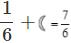# Test: Fraction - 3

## 20 Questions MCQ Test Mathematics (Maths) for Class 4 | Test: Fraction - 3

Description
This mock test of Test: Fraction - 3 for Class 4 helps you for every Class 4 entrance exam. This contains 20 Multiple Choice Questions for Class 4 Test: Fraction - 3 (mcq) to study with solutions a complete question bank. The solved questions answers in this Test: Fraction - 3 quiz give you a good mix of easy questions and tough questions. Class 4 students definitely take this Test: Fraction - 3 exercise for a better result in the exam. You can find other Test: Fraction - 3 extra questions, long questions & short questions for Class 4 on EduRev as well by searching above.
QUESTION: 1

### Mohit has some animal cards as shown. What fraction of the cards is cat card?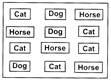Solution:

Total number of cards in the bag = 12 Number of cat cards = 5
∴ Required fraction = 5/12

QUESTION: 2

### Kriti puts the tiles shown below into an empty bag and mixed them up.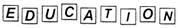What fraction of letters on tiles are vowels?

Solution:

Total number of letters in the word EDUCATION = 9 Number of vowels = 5
∴ Required fraction = 5/9

QUESTION: 3

### Harshit won exactly 3/5 of the ribbons for 1st place Which of the following could be the group of ribbons Harshit won?

Solution:

Harshit won exactly - of the ribbons for the 1st place means Harshit won 3 ribbons for 1st place out of 5 ribbons.

QUESTION: 4

The given list shows the names of the students who are in the Sports Club. Students in the Sports Club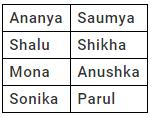One student will be chosen at random. What fraction of names are starting with alphabet S?

Solution:

Total number of students = 8 Number of students whose name starts with 'S' = 4
∴ Required fraction = 4/8

QUESTION: 5

Sonal bought 9 eggs out of which 6 are bad. What fraction of eggs are good?

Solution:

Total number of eggs Sonal bought = 9 Number of bad eggs = 6
∴ Number of good eggs = 9 − 6 = 3 So, required fraction = 3/9 = 1/3

QUESTION: 6

Shaded fraction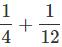is represented by which one of the following figures?

Solution: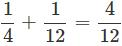which is equal to shaded fraction of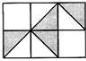QUESTION: 7

In an aquarium, there are 4 cat fish, 5 gold fish and 1 star fish. Which of the following statements about gold fish is true?

Solution:

Total number of fish in the aquarium = 10 Fraction of gold fish = 5/10 = 1/2 ⇒ Half of the fish in aquarium are gold fish.

QUESTION: 8

Which number should come in place of ??' so that sum of each arm is same?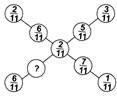Solution:

We have,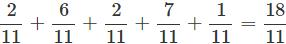Let the missing number be X. According to question,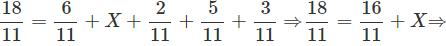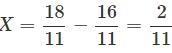QUESTION: 9

What is the value of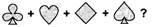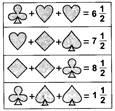Solution:

We have,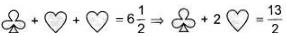...(i)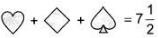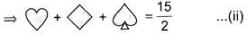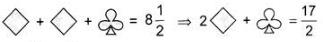...(iii)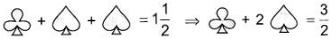...(iv)
Adding (i), (ii), (iii) and (iv), we get,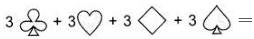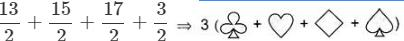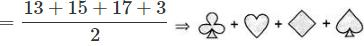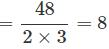QUESTION: 10

A fractional part of the set of stars is shaded.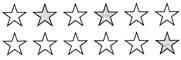Which of the following set of circles represents the same fraction?

Solution:

Total number of stars =12 Number of shaded stars = 3
So, fraction of shaded stars =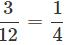which is equal to the shaded fraction of

QUESTION: 11

What is the sum of 4/13, 3/13 and 5/13?

Solution: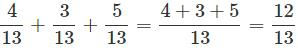QUESTION: 12

What is the difference between 12/19 and 4/19?

Solution:
QUESTION: 13

What should be added to 11/17 to make it 15/17?

Solution:
QUESTION: 14

Identify an example of proper fraction.

Solution:

fraction where the numerator (the top number) is less than the denominator (the bottom number).

Example: 1/4 (one quarter) and 5/6 (five sixths) are proper fractions.

QUESTION: 15

How can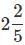be represented?

Solution:
QUESTION: 16

A How many one thirds are there in 72?

Solution:

The number of one thirds in 72 = 72 × 3 = 216.

QUESTION: 17

Which of the following fractions is less than 1/8?

Solution: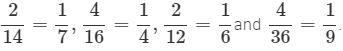Comparing the fractions, we get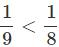QUESTION: 18

What fraction of the given figure is shaded?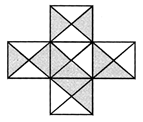Solution:

Total no. of equal parts in the given figure = 20. No. of shaded parts = 10
∴ The required fraction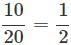QUESTION: 19

Observe the given sum.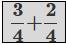What type of fraction is it?

Solution:

5/4 is an improper fraction.

QUESTION: 20

If=7/6 and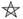+1/2 = 2/3, find the value of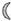.

Solution:

Given: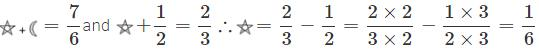Since,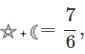we get